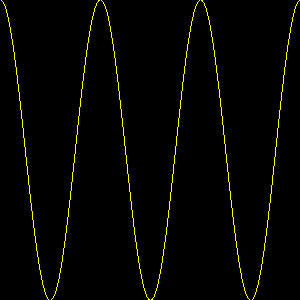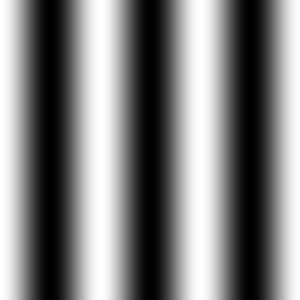# Image Profile

Hi There,

I want to implement in my application the possibility to have an xy plot of the intensity values of an image along the X or the Y axis. I have tried to draw vtkLineSource on top of my image and extract the intensity values with vtkImageProbeFilter but this approach doesn’t work. Do you have Any suggestion on how to implement this?

It should definitely be possible to do this with either vtkImageProbeFilter or with vtkProbeFilter. Here’s a short checklist:

1. Make sure the line has enough points, e.g. SetResolution(100)
2. Make sure the Bounds of the line are within the Bounds of the image

For the latter, note that both vtkPolyData (the line) and vtkImageData (the image) have a GetBounds() method, so the bounds check should be easy to do.

Are you working with 3D images or 2D images? For 2D, the line points must lie within the plane of the image.

Once the intensity values are attached to the line, it should be possible to use vtkWarpScalar to turn the line into a profile that can be rendered on the image.

Dear David,

Thanks.

How many point the line should have? Same size of the image?
I have checked that the bounds of the line and the 2D mage are the same.

Following an extract of my code

``````vtkImageData* data = myfits->GetOutput();
double* range = data->GetPointData()->GetScalars()->GetRange();
double p0_x = {1.0, world_coord, 1};
double p1_x = {double(myfits->GetNaxes(0)), world_coord, 1};

vtkNew<vtkLineSource> lineSource_x;
lineSource_x->SetPoint1(p0_x);
lineSource_x->SetPoint2(p1_x);
lineSource_x->SetResolution(100);
lineSource_x->Update();

vtkNew<vtkImageProbeFilter> probe2;
probe2->SetInputConnection(lineSource_x->GetOutputPort());
probe2->SetSourceConnection(myfits->GetOutputPort());
probe2->Update();
``````

Can you help me on the next steps to follow?

Yes, one point per image voxel will make a good profile. You can use `SetResolution(size-1)` to get the correct number of points. The resolution is the number of segments, which is one less than the number of points.

Is the z coordinate of your image always 1, and not 0? To me, it seems strange that your coordinates start at 1 instead of 0, so I expected something like this:

``````double p0_x = {0, world_coord, 0};
double p1_x = {double(myfits->GetNaxes(0))-1, world_coord, 0};
``````

As a next step, you can try putting the output of `probe2` into `vtkWarpScalar`, and then render the output of `vtkWarpScalar`. If everything works, it will look like a profile.

Thanks,

I have added the following code

``````   vtkNew<vtkWarpScalar> warp;
warp->SetInputConnection(probe2->GetOutputPort());

vtkNew<vtkDataSetMapper> mapper;
mapper->SetInputConnection(warp->GetOutputPort());
mapper->SetScalarRange(0, 255);
vtkNew<vtkActor> profile;
profile->SetMapper(mapper);
``````

But the result is empty

If I use the following code

``````vtkSmartPointer<vtkXYPlotActor> profile = vtkSmartPointer<vtkXYPlotActor>::New();
profile->GetPositionCoordinate()->SetValue(0.05, 0.05, 0);
profile->GetPosition2Coordinate()->SetValue(0.95, 0.95, 0);
profile->SetXValuesToNormalizedArcLength();
``````

The profile is not the one I expect

Thanks

I still suspect that your line is not placed correctly, and is therefore not within the image bounds. Have you checked the numerical values of the scalars that are produced by the probe filter?

Here is a small Python example that I wrote for image probing:

``````import vtkmodules.all as vtk

source = vtk.vtkImageSinusoidSource()
source.SetDirection(1.0, 0.0, 0.0)
source.SetPeriod(100)
source.SetWholeExtent(0, 299, 0, 299, 0, 0)
source.SetAmplitude(1.0)

line = vtk.vtkLineSource()
line.SetPoint1(0.0, 100.0, 0.0)
line.SetPoint2(299.0, 100.0, 0.0)
line.SetResolution(299)

probe = vtk.vtkImageProbeFilter()
probe.SetSourceConnection(source.GetOutputPort())
probe.SetInputConnection(line.GetOutputPort())
probe.Update()

profile = probe.GetOutput().GetPointData().GetScalars()
n = profile.GetNumberOfValues()
print("Profile Data:")
for i in range(n):
print(profile.GetValue(i))

print("Image Bounds:")
source.Update()
print(source.GetOutput().GetBounds())
print("Line Bounds:")
line.Update()
print(line.GetOutput().GetBounds())

warp = vtk.vtkWarpScalar()
warp.SetInputConnection(probe.GetOutputPort())
warp.SetNormal(0.0, 1.0, 0.0)
warp.SetScaleFactor(150.0)

mapper = vtk.vtkDataSetMapper()
mapper.SetInputConnection(warp.GetOutputPort())
mapper.ScalarVisibilityOff()

actor = vtk.vtkActor()
actor.SetMapper(mapper)
actor.GetProperty().SetColor(1.0, 1.0, 0.0)

renderer = vtk.vtkRenderer()

renwin = vtk.vtkRenderWindow()

iren = vtk.vtkRenderWindowInteractor()
renwin.SetInteractor(iren)
iren.Initialize()
iren.Start()

grab = vtk.vtkWindowToImageFilter()
grab.SetInput(renwin)
grab.Update()

writer = vtk.vtkPNGWriter()
writer.SetInputData(grab.GetOutput())
writer.SetFileName("profile.png")
writer.Write()
``````

It produces the following profile:from the following image:Thanks. I am now able to plot the profile. The issue was solved passing my data to the probe filter in the following way:

``````    probe_y->SetSourceData(myfits->GetOutput());

``````